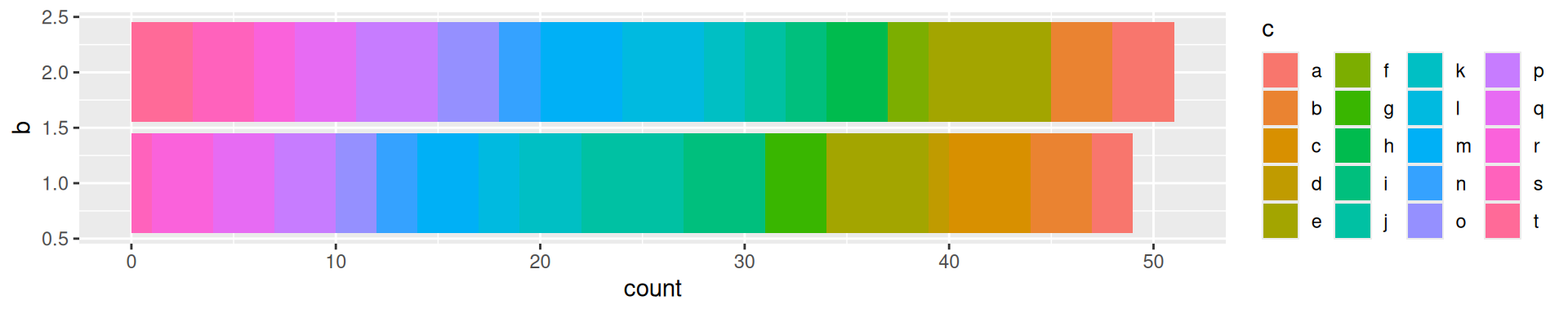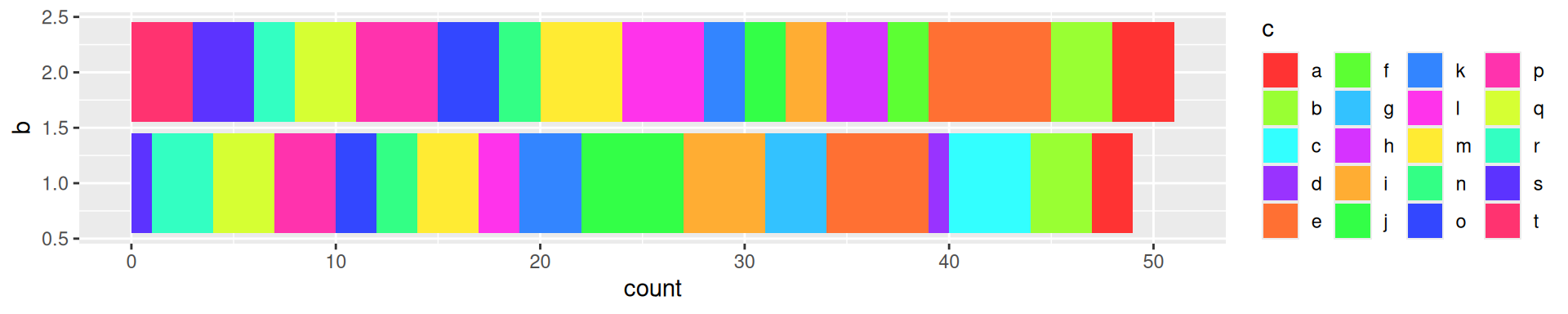# R

## Details to remember

• Use rbindlist from data.table package for a memory-optimized and faster do.call(rbind, list(..)).
• Use system2 instead of system to run a command. It’s more portable apparently.
• Get RPubs working with options(rpubs.upload.method = "internal").
• Operations on dates using strptime(x, "%a %b %d %T PST %Y'") and difftime and then as.Double(d, units="hours").

### Order in condition assessment

Using & and | operators, R tries all the conditions and then performs the operations. However, sometimes we would like a smarter sequential assessment for AND. For example, we get an error if we run:

x = NULL
if(!is.null(x) & x>10) message("so big !")

That’s because it tries to do x>10 when x is NULL. Here, what we want is &&:

x = NULL
if(!is.null(x) && x>10) message("so big !")
x = 17
if(!is.null(x) && x>10) message("so big !")

Now it won’t try to do x<10 if !is.null(x) is not true (because what’s the point, anything “False AND …” is for sure False). Caution, && doesn’t work on vectors (it will only test the first element).

## Graphs

• pdf("g.pdf", 9, 7)
• png("g.png", 1300, 1000, res=200)
• options(device=function() pdf(width=9, height=7)) to set the default device (e.g. remote graphs).

### Palette with many colors

When I need many colors and want to distinguish consecutive classes (e.g. bar graphs or overlapping clusters), I use an interleaved rainbow-like palette:

interk <- function(x, k=4){ # Interleaves elements in x
idx = unlist(lapply(1:k, function(kk) seq(kk, length(x), k)))
x[idx]
}
pal = interk(rainbow(20, s=.8), 5)

For example:

ggplot(bar.df, aes(x=x, fill=c)) + geom_bar() + coord_flip() +
guides(fill=guide_legend(ncol=4))ggplot(bar.df, aes(x=x, fill=c)) + geom_bar() + coord_flip() +
guides(fill=guide_legend(ncol=4)) +
scale_fill_manual(values=pal)### ggplot2 tricks

• To plot a density distribution without the x-axis line, use stat_density(geom="line") (and eventually position="dodge" if plotting several groups).
• To override the legend’s aes: guides(colour=guide_legend(override.aes=list(alpha=1)))
• Multi-column legends: guides(fill=guide_legend(ncol=4))

### Multi-panel ggplots

In general we can use grid.arrange. For example:

p1 = ggplot(...) + ...
p2 = ggplot(...) + ...
p3 = ggplot(...) + ...
grid.arrange(p1, p2, p3, heights=c(2,1), layout_matrix=rbind(c(1,1), 2:3))

#### Aligning the x-axis

Sometimes we might want to have two graphs, one on top of the other, with their x-axis aligned.

One easy way is to use the tracks function in the ggbio package. However, I don’t really like this package because it sometimes conflicts with ggplot2 (boo!) and you end up having to specify ggplot2:: to the functions to avoid obscure errors.

I found another way on the internet:

library(ggplot2)
library(gridExtra)
p1 <- ggplot(...
p2 <- ggplot(...
p1 <- ggplot_gtable(ggplot_build(p1))
p2 <- ggplot_gtable(ggplot_build(p2))
maxWidth = unit.pmax(p1$widths[2:3], p2$widths[2:3])
p1$widths[2:3] <- maxWidth p2$widths[2:3] <- maxWidth
grid.arrange(p1, p2, heights = c(3, 2))

### Change font in ggplot2

library(extrafont)
font_import(pattern='Comic')
qplot(x=rnorm(100)) + geom_histogram() + theme(text=element_text(family="Comic Sans MS")) + ggtitle('Ouch')

fonts() to check which fonts are imported by extrafont, names(pdfFonts()) to list the fonts available (loaded).

More in this blog post.

### Waffle graphs

waffle package provides a waffle and iron function. For example:

iron(
waffle(c(thing1=0, thing2=100), rows=5, keep=FALSE, size=0.5, colors=c("#af9139", "#544616")),
waffle(c(thing1=25, thing2=75), rows=5, keep=FALSE, size=0.5, colors=c("#af9139", "#544616"))
)

## Rmarkdown

• \ to force a line break and add vertical spacing (e.g. in slides).

To define knitr parameters, I add a chunk at the beginning of the Rmarkdown document. For example:

{r include=FALSE}
knitr::opts_chunk$set(echo=FALSE, message=FALSE, warning=FALSE, fig.width=10)  ### Beamer presentation Some useful options to put in the YAML header: title: The Title subtitle: The Subtitle author: Jean Monlong date: 11 Oct. 2016 output: beamer_presentation: slide_level: 2 fig_width: 7 includes: in_header: header.tex toc: true dev: png keep_tex: true • slide_level defines the header level to be considered as a new slide. To add slide count I put this on the header.tex: \setbeamertemplate{navigation symbols}{} \setbeamertemplate{footline}[page number] #### Wide tables To resize wide tables I use a hook that surround a chunk with \resizebox command, defined in the non-included chunk: {r, include=FALSE} knitr::knit_hooks$set(resize = function(before, options, envir) {
if (before) {
return('\\resizebox{\\textwidth}{!}{')
} else {
return('}')
}
})


## Wide table

{r, resize=TRUE}}
knitr::kable(matrix(rnorm(10),10,10), format='latex')


### Jekyll website

The Rmd files located in the _source folder get automatically compiled by servr package using this command:

Rscript -e "servr::jekyll(script='build.R', serve=FALSE)"

Note: I now use blogdown which automatically compile the R Markdown documents (every page is a R Markdown actually).

## data.table package

I’m more of a tidyverse person but for very large data the data.table package is more efficient.

tidyverse data.table
group_by(col1,col2) %>% summarize(nb=n()) dt[,.(nb=.N),by=.(col1,col2)]
group_by(col1,col2) %>% mutate(nb=n()) dt[,nb:=.N,by=.(col1,col2)]
filter(nb==2) dt[nb==2]

## Linux setup

Necessary to compile some R packages:

sudo apt-get install libxml2-dev libssl-dev libmariadbclient-dev libcurl4-openssl-dev

Related to XML, OpenSSL, MySQL, Curl respectively.